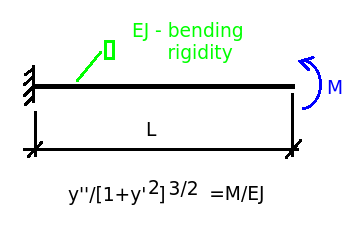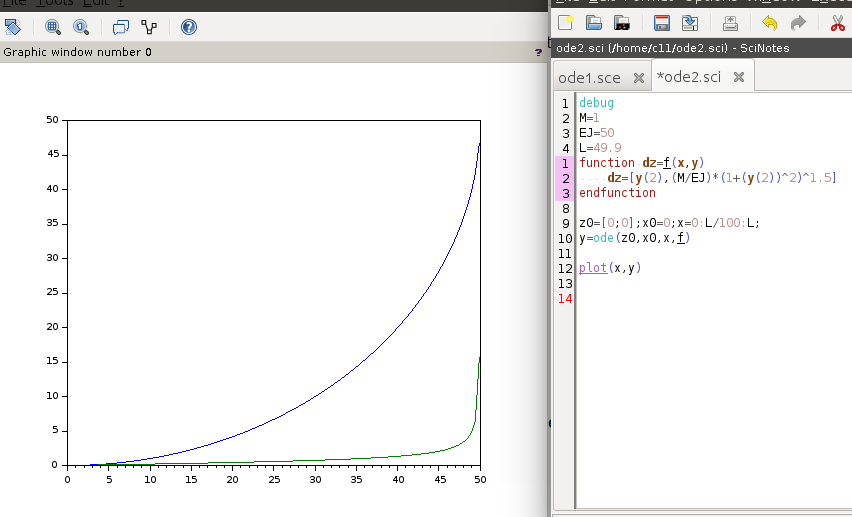### SciLab ODE second order

Example #1
Cantilever beam under the concentrated momentcode
_______________________________________

debug
M=1
EJ=50
L=49.9
function dz=f(x,y)
dz=[y(2),(M/EJ)*(1+(y(2))^2)^1.5]
endfunction

z0=[0;0];x0=0;x=0:L/100:L;
y=ode(z0,x0,x,f)

plot(x,y)

_______________________________________

result is 1/4 of circle (curve of constant curvature - blue)
'y' is the vector in the code above, it is why we have 2 curves (solution and derivative)